# MP Board Class 10th Maths Solutions Chapter 6 Triangles Ex 6.3

In this article, we will share MP Board Class 10th Maths Book Solutions Chapter 6 Triangles Ex 6.3 Pdf, These solutions are solved subject experts from the latest edition books.

## MP Board Class 10th Maths Solutions Chapter 6 Triangles Ex 6.3

Question 1.
State which pairs of triangles in the given figures are similar. Write the similarity criterion used by you for answering the question and also write the pairs of similar triangles in the symbolic form.Solution:
(i) In ∆ABC and ∆PQR,
We have : ∠A = ∠P = 60°
∠B = ∠Q = 80°
∠C = ∠R = 40°
∴ The corresponding angles are equal.
∴ Using the AAA similarity rule,
∆ABC ~ ∆PQR

(ii) In ∆ABC and ∆QRP,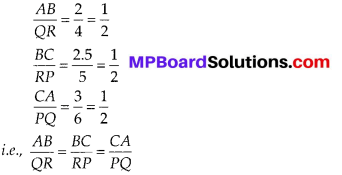i.e., Using the SSS similarity, ∆ABC ~ ∆QRP

(iii) In ∆LMP and ∆DEF,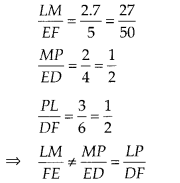∴ Triangles are not similar,

(iv) In ∆MNL and ∆QPR,
$$\frac{M L}{Q R}=\frac{M N}{Q P}$$ [$$\frac{1}{2}$$ each]
and ∠NML = ∠PQR
∴ Using SAS similarity, we have
∆MNL ~ ∆QPR.

(v) In ∆ABC and ∆FDE,
$$\frac{A B}{D F}=\frac{B C}{E F}$$ [$$\frac{1}{2}$$ each]
Now, angle between DF and EF is 80°. But angle between AB and BC is unknown.
∴ Triangles are not similar.

(vi) In ∆DEF and ∆PQR,
∠D = ∠P = 70°
[∵ ∠P = 180° – (80° + 30°) = 180° – 110° = 70°]
∠E = ∠Q = 80°
∠F = ∠R = 30° [∵∠F = 180°]
∴ Using the AAA similarity rule,
∆DFE ~ ∆PRQ.

Question 2.
In the figure, ∆ODC ~ ∆OBA, ∠BOC = 125° and ∠CDO = 70°. Find ∠DOC, ∠DCO and ∠OAB.Solution:
We have, ∠BOC = 125° and ∠CDO = 70°
since, ∠DOC + ∠BOC = 180° [Linear pair]
⇒ ∠DOC = 180° – 125° = 55° ……………… (1)
In ∆DOC,
Using the angle sum property for ∆ODC, we get
∠DOC + ∠ODC + ∠DCO = 180°
⇒ 55° + 70° + ∠DCO = 180°
⇒ ∠DCO = 180° – 55° – 70° = 55°
Again,
∠DOC = ∠BOA ……………. (2) [vertically opposite angles]
and ∠OCD = ∠OAB = 55° ………….. (3)
[corresponding angles of similar triangles]
Thus, from (1), (2) and (3)
∠DOC = 55°, ∠DCO = 55° and ∠OAB = 55°.Question 3.
Diagonals AC and BD of a trapezium ABCD with AB || DC intersect each other at the point O. Using a similarity criterion for two triangles, show that $$\frac{O A}{O C}=\frac{O B}{O D}$$.
Solution:
We have a trapezium ABCD in which AB || DC. The diagonals AC and BD intersect at O.In ∆OAB and ∆OCD,
∠OBA = ∠ODC (Alternate angles)
and ∠OAB = ∠OCD(Alternate angles)
Using AA similarity rule, ∆OAB ~ ∆OCD
So, $$\frac{O B}{O D}=\frac{O A}{O C}$$ (Ratios ot corresponding sides of the similar triangles)
⇒ $$\frac{O A}{O C}=\frac{O B}{O D}$$

Question 4.
In the figure, $$\frac{Q R}{Q S}=\frac{Q T}{P R}$$ and ∠1 = ∠2. show that ∆PQS ~ ∆TQR.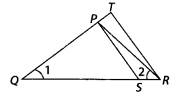Solution:
In ∆PQR
∵ ∠1 = ∠2 [Given]
∴ PR = QP ……………… (1)
[∵ In a ∆ sides opposite to equal angles are equal]and ∠SQP = ∠RQT = ∠1
Now, using SAS similarity rule,
∆PQS ~ ∆TQR.

Question 5.
S and T are points on sides PR and QR of ∆PQR such that ∠P = ∠RTS. Show that ∆RPQ ~ ∆RTS.
Solution:
In ∆PQR,
T is a point on QR and S is a point on PR such that ∠RTS = ∠P.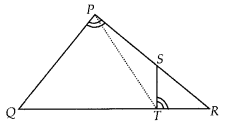Now in ∆RPQ and ∆RTS,
∠RPQ = ∠RTS [Given]
∠PRQ = ∠TRS [Common]
Using AA similarity, we have ∆RPQ ~ ∆RTS.

Question 6.
In the figure, if ∆ABE ≅ ∆ACD, show that ∆ADE ~ ∆ABC.
Solution:
We have,
∆ABE ≅ ∆ACD
Their corresponding parts are equal, i.e.,
AB = AC, AE = AD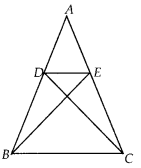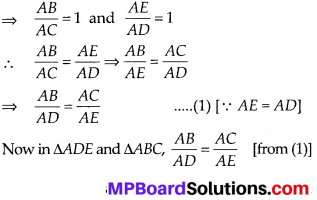and ∠DAE = ∠BAC (common)
∴ Using the SAS similarity, we have ∆ADE ~ ∆ABC.Question 7.
In the figure, altitudes AD and CE of ∆ABC intersect each other at the point P. Show that:
(i) ∆AEP ~ ∆CDP
(ii) ∆ABD ~ ∆CBE
(iv) ∆PDC ~ ∆BEC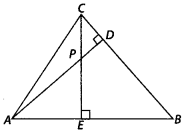Solution:
We have a ∆ABC in which altitude AD and CE intersect each other at P.
⇒ ∠D = ∠E = 90° …………. (1)
(i) In ∆AEP and ∆CDP,
∠AEP = ∠CDP [From (1)]
∠EPA = ∠DPC [Vertically opp. angles]
∴ Using AA similarity, we get
∆AEP ~ ∆CDP

(ii) In ∆ABD and ∆CBE,
Also, ∠ABD = ∠CBE [Common]
Using A A similarity, we have
∆ABD ~ ∆CBE

∵ ∠AEP = ∠ADB [From (1)]
Also, ∠EAP = ∠DAB [Common]
∴ Using AA similarity, we have

(iv) In ∆PDC and ∆BEC,
∵ ∠PDC = ∠BEC [From (1)]
Also, ∠DCP = ∠ECB [Common]
∴ Using AA similarity, we have
∆PDC ~ ∆BEC

Question 8.
E is a point on the side AD produced of a parallelogram ABCD and BE intersects CD at F. Show that ∆ABE ~ ∆CFB.
Solution:
We have a parallelogram ABCD in which AD is produced to E and BE is joined such that BE intersect CD at F.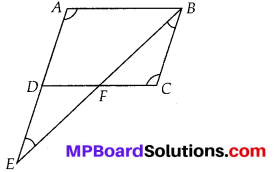Now, in ∆ABE and ∆CFB
∠BAE = ∠FCB [Opp. angles of a || gm are always equal]
∠AEB = ∠CBF [∵ Parallel sides are intersected by the transversal BE]
Now, using AA similarity, we have ∆ABE ~ ∆CFB.

Question 9.Prove that:
(i) ∆ABC ~ ∆AMP
(ii) $$\frac{C A}{P A}=\frac{B C}{M P}$$
Solution:
We have ∆ABC, right angled at B and ∆AMP, right angled at M.
∴ ∠B = ∠M = 90°
(i) In ∆ABC and ∆AMP,
∠ABC = ∠AMP [From (1)]
and ∠BAC = ∠MAP [Common]
∴ Using AA similarity, we get
∆ABC ~ ∆AMP

(ii) ∵ ∆ABC ~ ∆AMP [As proved above]
∴ Their corresponding sides are proportional.
⇒ $$\frac{C A}{P A}=\frac{B C}{M P}$$

Question 10.
CD and GH are respectively the bisectors of ∠ACB and ∠EGF such that 0 and H lie on sides AB and FE of ∆ABC and ∆EFG respectively. If ∆ABC ~ ∆FEG, show that:
CD AC
(i) $$\frac{C D}{G H}=\frac{A C}{F G}$$
(ii) ∆DCB ~ ∆HGE
(iii) ∆DCA ~ ∆HGF
Solution:
We have, two similar ∆ABC and ∆FEG such that CD and GH are the bisectors of ∠ACB and ∠FGE respectively.(i) In ∆ACD and ∆FGH,
Since ∆ABC ~ ∆FEG
∠A = ∠F …………….. (1)
and ∠ACB = ∠FGE ⇒ $$\frac{1}{2}$$ ∠ACB = $$\frac{1}{2}$$ ∠FGE
⇒ ∠ACD = ∠FGH ……………. (2)
From (1) and (2),
∆ACD ~ ∆FGH [AA similarity]
∴ Their corresponding sides are proportional,
∴ $$\frac{C D}{G H}=\frac{A C}{F G}$$

(ii) In ∆DCB and ∆HGE,
Since ∆ABC ~ ∆FEG
⇒ ∠B = ∠E …………….. (1)
and ∠ACB = ∠FGE
∴ $$\frac{1}{2}$$∠ACB = $$\frac{1}{2}$$∠FGE
⇒ ∠DCB = ∠HGE ……………. (2)
From (1) and (2),
∆DCB ~ ∆HGE [AA similarity]

(iii) From (i) part, we get
∆ACD ~ ∆FGH
⇒ ∆DCA ~ ∆HGFQuestion 11.
In the figure, E is a point on side CB produced of an isosceles triangle ABC with AB = AC. If AD⊥BCand EF⊥AC, prove that ∆ABD ~ ∆ECF.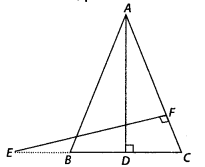Solution:
We have an isosceles ∆ABC in which
AB = AC.
⇒ Angles opposite to them are equal
∠ACB = ∠ABC ……………. (1)
In ∆ABD and ∆ECF,
∠ECF = ∠ABD [from (1)]
⇒ ∠ADB = ∠EFC = 90°
∴ ∆ABD ~ ∆ECF [AA similarity]

Question 12.
Sides AB and BC and median AD of a triangle ABC are respectively proportional to sides PQ and QR and median PM of ∆PQR (see figure). Show that ∆ABC ~ ∆PQR.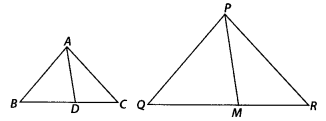Solution:
We have ∆ABC and ∆PQR in which AD and PM are medians corresponding to sides BC and QR respectively such that
$$\frac{A B}{P Q}=\frac{B C}{Q R}=\frac{A D}{P M}$$∴ Using SSS similarity, we have ∆ABD ~ ∆PQM
∴ Their corresponding angles are equal.
⇒ ∠ABD = ∠PQM ⇒ ∠ABC = ∠PQR
Now, in ∆ABC and ∆PQR, $$\frac{A B}{P Q}=\frac{B C}{Q R}$$ …………. (1)
Also, ∠ABC = ∠PQR …………… (2)
From (1) and (2),
∆ABC ~ ∆PQR. (SAS similarity)

Question 13.
O is a point on the side BC of a triangle ABC such that ∠ADC = ∠BAC. Show that CA2 = CB CD.
Solution:
We have a ∆ABC and a point D on its side BC such that ∠ADC = ∠BAC.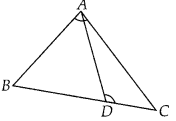and ∠BCA = ∠DCA [Common]
Using AA similarity, we have
∴ Their corresponding sides are proportional.
⇒ $$\frac{C A}{C D}=\frac{C B}{C A}$$
⇒ CA × CA = CB × CD
⇒ CA2 = CB × CD

Question 14.
Sides AB and AC and median AD of a triangle ABC are respectively proportional to sides PQ and PR and median PM of another triangle PQR. Show that ∆ABC ~ ∆PQR.
Solution:
Given : ∆ABC and ∆PQR in which AD and PM are medians.
Also, $$\frac{A B}{P Q}=\frac{A C}{P R}=\frac{A D}{P M}$$ …………….. (1)
To Prove: ∆ABC ~ ∆PQR
Construction: Produce AD to E and PM to N such that AD = DE and PM = MN. Join BE, CE, QN and RN.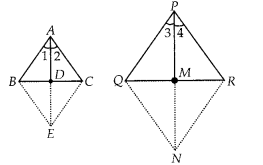Proof: Quadrilaterals ABEC and PQNR are parallelograms, since their diagonals bisect each other at point D and M respectively.
⇒ BE = AC and QN = PR⇒ ∆ABE ~ ∆PQN ⇒ ∠1 = ∠3 …………. (4)
Similarly, we can prove
⇒ ∆ACE ~ ∆PRN ⇒ ∠2 = ∠4 …………….. (5)
From (4) and (5)
⇒ ∠1 + ∠2 = ∠3 + ∠4
⇒ ∠A = ∠P ………….. (6)
Now, in ∆ABC and ∆PQR, we have
$$\frac{A B}{P Q}=\frac{A C}{P R}$$ [From (1)]
and ∠A = ∠P [From (6)]
∴ ∆ABC ~ ∆PQRQuestion 15.
A vertical pole of length 6 m casts a shadow 4 m long on the ground and at the same time a tower casts a shadow 28 m long. Find the height of the tower.
Solution:
Let AB = 6 m be the pole and BC = 4 m be its shadow (in right ∆ABC), whereas DE and EE denote the tower and its shadow respectively.
∵ EF = Length of the shadow of the tower = 28 m
and DE = h = Height of the towerIn ∆ABC and ∆DEF, we have ∠B = ∠E = 90°
∠C = ∠F [∵ Angular elevation of the sun at the same time is equal]
∴ Using AA similarity, we have
∆ABC ~ ∆DEF
∴ Their sides are proportional i.e., $$\frac{A B}{D E}=\frac{B C}{E F}$$
⇒ $$\frac{6}{h}=\frac{4}{28}$$ ⇒ h = $$\frac{6 \times 28}{4}$$ = 42
Thus, the required height of the tower is 42 m.

Question 16.
If MD and PM are medians of triangles ABC and PQR, respectively where, ∆ABC ~ ∆PQR, prove that $$\frac{A B}{P Q}=\frac{A D}{P M}$$.
Solution:
We have ∆ABC ~ ∆PQR such that AD and PM are the medians corresponding to the sides BC and QR respectively.∵ ∆ABC ~ ∆PQR
And the corresponding sides of similar triangles are proportional.
∴ $$\frac{A B}{P Q}=\frac{B C}{Q R}=\frac{C A}{R P}$$ ……………. (1)
∵ Corresponding angles are also equal in two similar triangles.
∴ ∠A = ∠P, ∠B = ∠Q and ∠C = ∠R …………… (2)
Since, AD and PM are medians.
∴ BC = 2BD and QR = 2QM
∴ From (1) $$\frac{A B}{P Q}=\frac{2 B D}{2 Q M}=\frac{B D}{Q M}$$ ………… (3)
And ∠B = ∠Q ⇒ ∠ABD = ∠PQM
∴ From (3) and (4), we have
∆ABD ~ ∆PQM (SAS similarity)
∴ Their corresponding sides are proportional.
⇒ $$\frac{A B}{P Q}=\frac{A D}{P M}$$